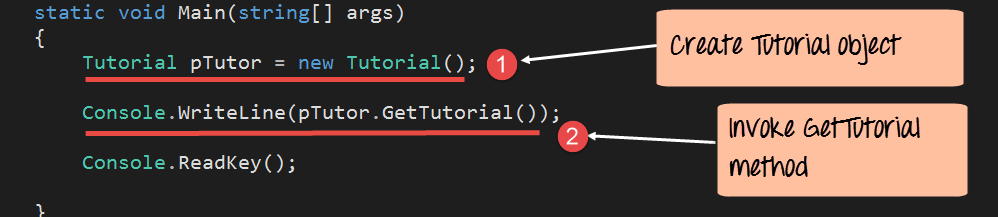In this tutorial, you will learn-

## Access Modifiers

Access Modifiers or Access Specifiers are used to define the visibility of a class property or method. There are times when you may not want other programs to see the properties or the methods of a class. In such cases, C# gives the ability to put modifiers on class properties and methods. The class modifiers can restrict access so that other programs cannot see the properties or methods of a class.

There are 3 types of access modifiers. They are explained below.

### Private

When this access specifier is attached to either a property or a method, it means that those members cannot be accessed from any external program.

Let's take an example and see what happens when we use the private access modifier.

Let's modify the current code in our Tutorial.cs file. In the SetTutorial method, let's change the public keyword to private.

Now let's switchover to our Program.cs file. You will notice that there is a red squiggly line under the SetTutorial method.

Since we have now declared the SetTutorial method as private in our Tutorial class, Visual Studio has detected this. It has told the user by highlighting it that now this method will not work from the Program.cs file.

### Public

When this access modifier is attached to either a property or a method, it means that those members can be accessed from any external program. We have already seen this in our earlier examples.

Since we have defined our methods as public in the Tutorial class, they can be accessed from the Program.cs file.

### Protected

When this access modifier is attached to either a property or a method, it means that those members can be accessed only by classes inherited from the current class. This will be explained in more detail in the Inheritance class.

## C# Constructor

Constructors are used to initializing the values of class fields when their corresponding objects are created. A constructor is a method which has the same name as that of the class. If a constructor is defined in a class, then it will provide the first method which is called when an object is created. Suppose if we had a class called Employee. The constructor method would also be named as Employee().

The following key things need to be noted about constructor methods

1. The access modifier for the constructor needs to be made as public.
2. There should be no return type for the constructor method.

Let's now see how we can incorporate the user of constructors in our code. We will use constructors to initialize the TutorialID and TutorialName fields to some default values when the object is created.

Step 1) The first step is to create the constructor for our Tutorial class. In this step, we add the below code to the Tutorial.cs file.

Code Explanation:-

1. We first add a new method which has the same name as that of the class. Because it is the same name as the class, C# treats this as a constructor method. So now whenever the calling method creates an object of this class, this method will be called by default.
2. In the Tutorial constructor, we are setting the value of TutorialID to 0 and TutorialName to "Default". So whenever an object is created, these fields will always have these default values.

Now let's switchover to our Program.cs file and just remove the line, which calls the SetTutorial method. This is because we want just to see how the constructor works.```using System;
using System.Collections.Generic;
using System.Linq;
using System.Text;
namespace DemoApplication
{
class Tutorial
{
public int TutorialID;
public string TutorialName;

public Tutorial()
{
TutorialID=0;
TutorialName="Default";
}
public void SetTutorial(int pID,string pName)
{
TutorialID=pID;
TutorialName=pName;
}
public String GetTutorial()
{
return TutorialName;
}

static void Main(string[] args)
{
Tutorial pTutor=new Tutorial();

Console.WriteLine(pTutor.GetTutorial());

}
}
}```

Code Explanation:-

1. The first step is to create an object for the Tutorial class. This is done via the 'new' keyword.
2. We use the GetTutorial method of the Tutorial class to get the TutorialName. This is then displayed to the console via the Console.WriteLine method.

If the above code is entered properly and the program is executed, the following output will be displayed.

Output:

From the output, we can see that the constructor was indeed called and that the value of the TutorialName was set to "Default".

Note: Here the value "default" is fetched from the constructor.

Summary

• Access Modifiers or Access Specifiers are used to define the visibility of a class property or method.
• A constructor is used to initialize the fields of a class whenever an object is created.
• The constructor is a method which has the same names as the class itself.

YOU MIGHT LIKE: